Search results

# Annotation in React Diagram component

13 May 2021 / 21 minutes to read

`Annotation` is a block of text that can be displayed over a node or connector. Annotation is used to textually represent an object with a string that can be edited at runtime. Multiple annotations can be added to a node/connector.

## Create annotation

An annotation can be added to a node/connector by defining the annotation object and adding that to the annotation collection of the node/connector. The `content` property of annotation defines the text to be displayed. The following code illustrates how to create a annotation.

Source
Preview
index.tsx
``````import * as React from "react";
import * as ReactDOM from "react-dom";
import {
Diagram,
DiagramComponent,
NodeModel
} from "@syncfusion/ej2-react-diagrams";
let diagramInstance: DiagramComponent;

// A node is created and stored in nodes array.
let node: NodeModel[] = [{
// Position of the node
offsetX: 100,
offsetY: 100,
// Size of the node
width: 100,
height: 100,
style: {
fill: '#6BA5D7',
strokeColor: 'white'
},
// Sets the Annotation for the Node
annotations: [{
// Sets the text to be displayed
content: 'Annotation'
}]
}];

let connector: connectorModel[] = [{
sourcePoint: {
x: 300,
y: 100
},
targetPoint: {
x: 400,
y: 300
},
type: 'Orthogonal',
style: {
strokeColor: '#6BA5D7'
},
// Sets the Annotation for the Connector
annotations: [{
// Sets the text to be displayed
content: 'Annotation'
}]
}];

// initialize Diagram component

ReactDOM.render( <DiagramComponent id = "diagram"
width = {
'100%'
}
height = {
'600px'
}
nodes = {
node
}
connectors = {
connector
}
// render initialized Diagram
/>,   document.getElementById("diagram") );``````

• Annotations can be added at runtime by using the client-side method `addLabels`. The following code illustrates how to add a annotation to a node.
• The annotation’s `ID` property is used to define the name of the annotation and its further used to find the annotation at runtime and do any customization.
Source
Preview
index.tsx
``````import * as React from "react";
import * as ReactDOM from "react-dom";
import {
Diagram,
NodeModel,
DiagramComponent,
ShapeAnnotationModel
} from "@syncfusion/ej2-react-diagrams";

// A node is created and stored in nodes array.
let node: NodeModel[] = [{
// Position of the node
offsetX: 250,
offsetY: 250,
// Size of the node
width: 100,
height: 100,
style: {
fill: '#6BA5D7',
strokeColor: 'white'
},
}];
let diagramInstance: DiagramComponent;

let annotation: ShapeAnnotationModel[] = [{
id: 'label1',
content: 'Annotation'
}];

// initialize Diagram component
ReactDOM.render( <DiagramComponent id = "diagram" ref={diagram => (diagramInstance = diagram)}
width = {
'100%'
}
height = {
'600px'
}
nodes = {
node
}
created = {
() => {
//Method to add labels at run time
diagramInstance.dataBind();
}
}
// render initialized Diagram
/>,   document.getElementById("diagram") );``````

## Remove annotation

A collection of annotations can be removed from the node by using client-side method `removeLabels`. The following code illustrates how to remove a annotation to a node.

Source
Preview
index.tsx
``````import * as React from "react";
import * as ReactDOM from "react-dom";
import {
Diagram,
DiagramComponent,
NodeModel,
ShapeAnnotationModel
} from "@syncfusion/ej2-react-diagrams";

let diagramInstance: DiagramComponent;

// A node is created and stored in nodes array.
let node: NodeModel[] = [{
// Position of the node
offsetX: 250,
offsetY: 250,
// Size of the node
width: 100,
height: 100,
style: {
fill: '#6BA5D7',
strokeColor: 'white'
},
// Sets the annotation for the node
annotations: [{
content: 'Annotation'
}]
}];
// initialize Diagram component

ReactDOM.render( <DiagramComponent
id = "diagram"
ref={diagram => (diagramInstance = diagram)}
width = {
'100%'
}
height = {
'600px'
}
nodes = {
node
}
created = {
() => {
diagramInstance.nodes.annotations.content = 'Updated Annotation';
//Method to update the annotation at run time
diagramInstance.dataBind();
}
}
// render initialized Diagram
/>,   document.getElementById("diagram") );``````

## Update annotation at runtime

You can change any annotation properties at runtime and update it through the client-side method `dataBind`.

The following code example illustrates how to change the annotation properties.

Source
Preview
index.tsx
``````import * as React from "react";
import * as ReactDOM from "react-dom";
import {
Diagram,
DiagramComponent,
NodeModel,
ShapeAnnotationModel
} from "@syncfusion/ej2-react-diagrams";

let diagramInstance: DiagramComponent;

// A node is created and stored in nodes array.
let node: NodeModel[] = [{
// Position of the node
offsetX: 250,
offsetY: 250,
// Size of the node
width: 100,
height: 100,
style: {
fill: '#6BA5D7',
strokeColor: 'white'
},
// Sets the annotation for the node
annotations: [{
content: 'Annotation'
}]
}];
// initialize Diagram component

ReactDOM.render( < DiagramComponent id = "diagram" ref={diagram => diagramInstance = diagram}
width = {
'100%'
}
height = {
'600px'
}
nodes = {
node
}
// render initialized Diagram
/>,   document.getElementById("diagram") );
diagramInstance.nodes.annotations.content = 'Updated Annotation';
//Method to update the annotation at run time
diagramInstance.dataBind();``````

## Alignment

Annotation can be aligned relative to the node boundaries. It has `margin`, `offset`, horizontal, and vertical alignment settings. It is quite tricky when all four alignments are used together but gives more control over alignment.

## Offset

The offset property of annotation is used to align the annotations based on fractions. 0 represents top/left corner, 1 represents bottom/right corner, and 0.5 represents half of width/height.

Set the size for a nodes annotation by using `width` and `height` properties.

The following code shows the relationship between the annotation position (black color circle) and offset (fraction values).

Source
Preview
index.tsx
``````import * as React from "react";
import * as ReactDOM from "react-dom";
import {
Diagram,
DiagramComponent,
NodeModel,
ShapeAnnotationModel
} from "@syncfusion/ej2-react-diagrams";

// A node is created and stored in nodes array.
let node: NodeModel[] = [{
// Position of the node
offsetX: 250,
offsetY: 250,
// Size of the node
width: 100,
height: 100,
style: {
fill: '#6BA5D7',
strokeColor: 'white'
},
// Sets the annotation for the node
annotations: [{
// Sets the content for the annotation
content: 'Annotation',
//Sets the offset for the content
offset: {
x: 0,
y: 1
}
}]
}];

// initialize Diagram component

ReactDOM.render( <DiagramComponent id = "diagram"
width = {
'100%'
}
height = {
'600px'
}
nodes = {
node
}
// render initialized Diagram
/>,   document.getElementById("diagram") );``````

## Horizontal and vertical alignment

The `horizontalAlignment` property of annotation is used to set how the annotation is horizontally aligned at the annotation position determined from the fraction values. The `verticalAlignment` property is used to set how annotation is vertically aligned at the annotation position.

The following tables illustrates all the possible alignments visually with ‘offset (0, 0)‘.

Horizontal Alignment Vertical Alignment Output with Offset(0,0)
Left Top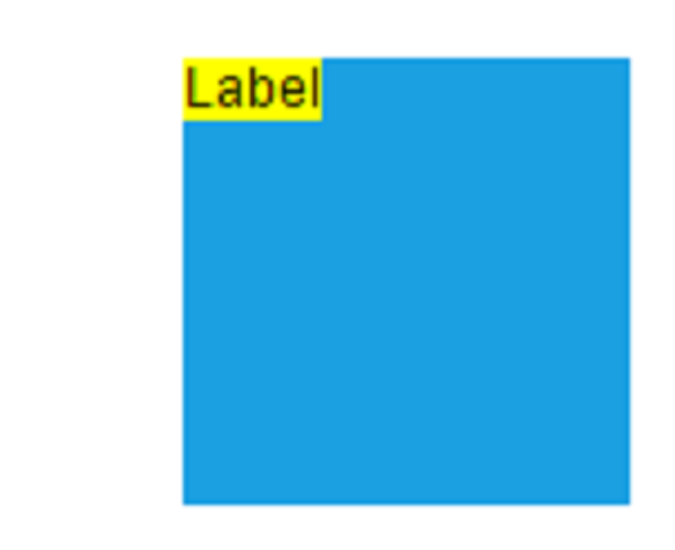Center Top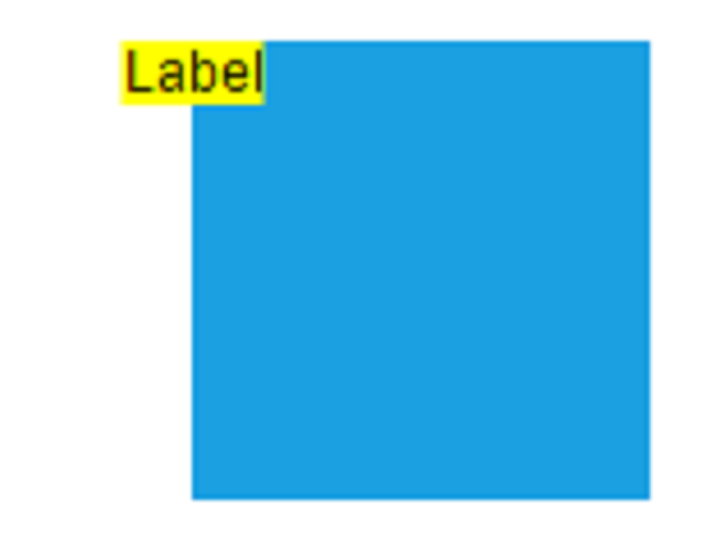Right Top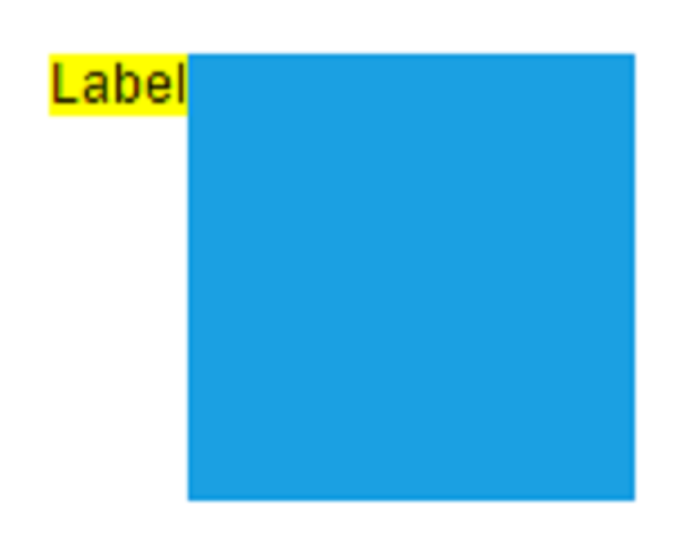Left Center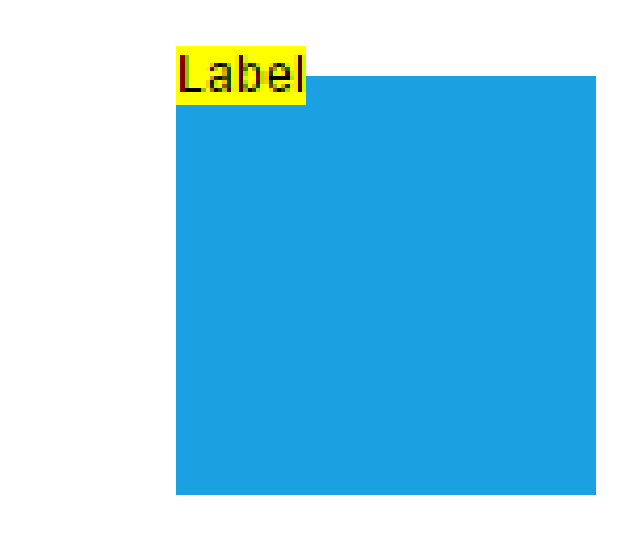Center Center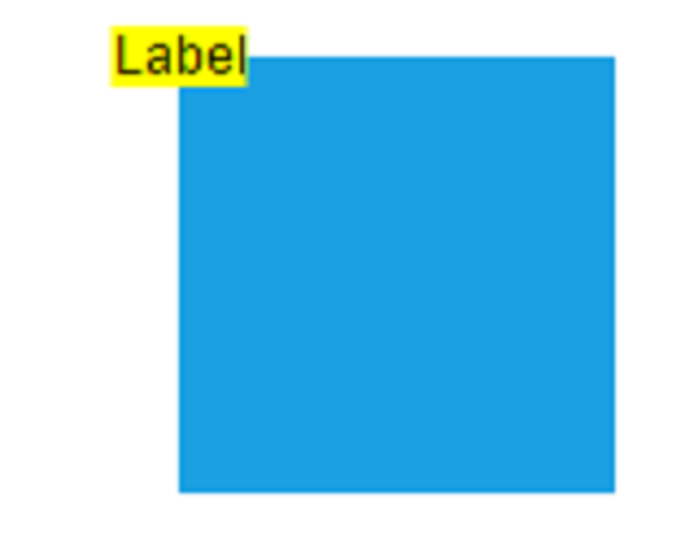Right Center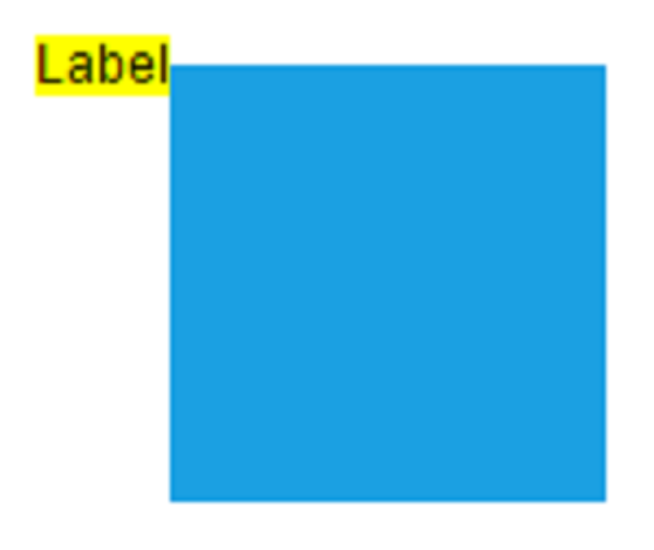Left Bottom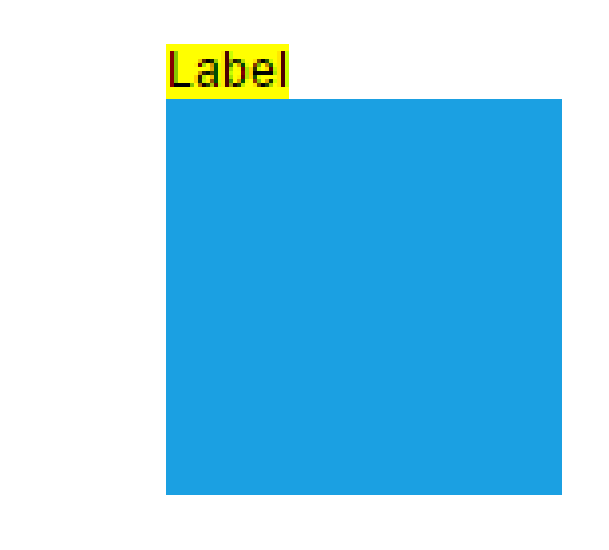Center Bottom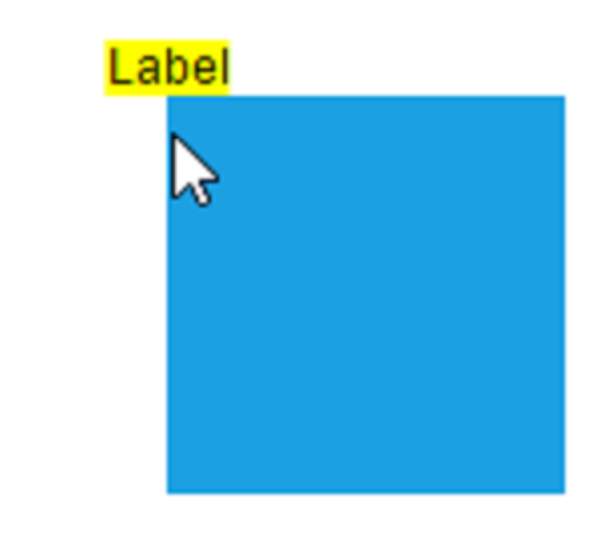Right Bottom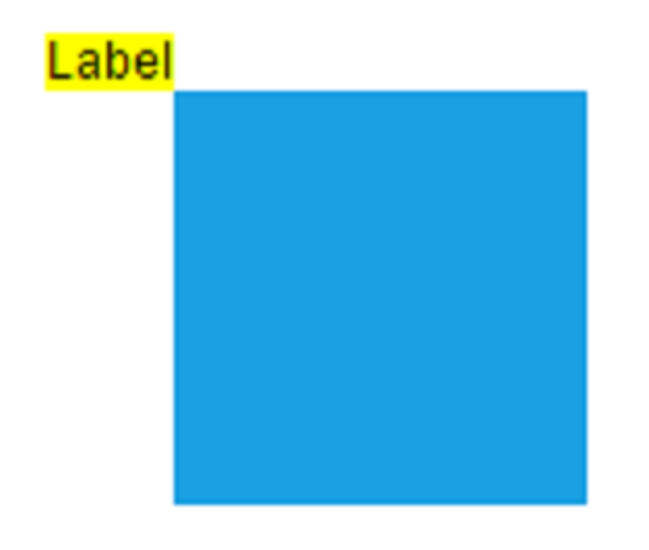The following codes illustrates how to align annotations.

Source
Preview
index.tsx
``````import * as React from "react";
import * as ReactDOM from "react-dom";
import {
Diagram,
DiagramComponent,
NodeModel,
ShapeAnnotationModel
} from "@syncfusion/ej2-react-diagrams";

// A node is created and stored in nodes array.
let node: NodeModel[] = [{
// Position of the node
offsetX: 250,
offsetY: 250,
// Size of the node
width: 100,
height: 100,
style: {
fill: '#6BA5D7',
strokeColor: 'white'
},
// Sets the annotation for the node
annotations: [{
content: 'Annotation',
// Sets the horizontal alignment as left
horizontalAlignment: 'Left',
// Sets the vertical alignment as Center
verticalAlignment: 'Center'
}]
}];

// initialize Diagram component

ReactDOM.render( <DiagramComponent id = "diagram"
width = {
'100%'
}
height = {
'600px'
}
nodes = {
node
}
// render initialized Diagram
/>,   document.getElementById("diagram") );``````

## Annotation alignment with respect to segments

The offset and alignment properties of annotation allows you to align the connector annotations with respect to the segments.

The following code example illustrates how to align connector annotations.

Source
Preview
index.tsx
``````import * as React from "react";
import * as ReactDOM from "react-dom";
import {
Diagram,
DiagramComponent,
NodeModel
} from "@syncfusion/ej2-react-diagrams";

// A node is created and stored in nodes array.
let node: NodeModel[] = [{
id: 'node1',
// Position of the node
offsetX: 100,
offsetY: 100,
// Size of the node
width: 100,
height: 100,
style: {
fill: '#6BA5D7',
strokeColor: 'white'
},
// Sets the annotation for the node
annotations: [{
}]
}, {
id: 'node2',
// Position of the node
offsetX: 300,
offsetY: 100,
// Size of the node
width: 100,
height: 100,
style: {
fill: '#6BA5D7',
strokeColor: 'white'
},
// Sets the annotation for the node
annotations: [{
}]
}];

let connector: connectorModel[] = [{
sourceID: 'node1',
targetID: 'node2',
type: 'Orthogonal',
style: {
strokeColor: '#6BA5D7',
strokeWidth: 2
},
// Sets the annotation for the connector
annotations: [{
content: '0',
// Sets the offset for the content
offset: 0
}, {
content: '1',
// Sets the offset for the content
offset: 1
}]
}];

// initialize Diagram component

ReactDOM.render( <DiagramComponent id = "diagram"
width = {
'100%'
}
height = {
'600px'
}
nodes = {
node
}
connectors = {
connector
}
// render initialized Diagram
/>,   document.getElementById("diagram") );``````

## Margin

`Margin` is an absolute value used to add some blank space in any one of its four sides. The annotations can be displaced with the margin property. The following code example illustrates how to align a annotation based on its `offset`, `horizontalAlignment`, `verticalAlignment`, and `margin` values.

Source
Preview
index.tsx
``````import * as React from "react";
import * as ReactDOM from "react-dom";
import {
Diagram,
DiagramComponent,
NodeModel
} from "@syncfusion/ej2-react-diagrams";

// A node is created and stored in nodes array.

let node: NodeModel[] = [{
id: 'node1',
// Position of the node
offsetX: 100,
offsetY: 100,
// Size of the node
width: 100,
height: 100,
style: {
fill: '#6BA5D7',
strokeColor: 'white'
},
// Sets the annotation for the connector
annotations: [{
// Sets the margin for the content
margin: {
top: 10
},
horizontalAlignment: 'Center',
verticalAlignment: 'Top',
offset: {
x: 0.5,
y: 1
}
}]
}];

// initialize Diagram component

ReactDOM.render( <DiagramComponent id = "diagram"
width = {
'100%'
}
height = {
'600px'
}
nodes = {
node
}
// render initialized Diagram
/>,   document.getElementById("diagram") );``````

## Text align

The `textAlign` property of annotation allows you to set how the text should be aligned (left, right, center, or justify) inside the text block. The following codes illustrate how to set textAlign for an annotation.

Source
Preview
index.tsx
``````import * as React from "react";
import * as ReactDOM from "react-dom";
import {
Diagram,
DiagramComponent,
NodeModel
} from "@syncfusion/ej2-react-diagrams";

// A node is created and stored in nodes array.

let node: NodeModel[] = [{
id: 'node1',
// Position of the node
offsetX: 100,
offsetY: 100,
// Size of the node
width: 100,
height: 100,
style: {
fill: '#6BA5D7',
strokeColor: 'white'
},
// Sets the annotation for the NOde
annotations: [{
content: 'Text align is set as Left',
// Sets the textAlign as left for the content
style: {
textAlign: 'Left'
}
}]
}];

// initialize Diagram component

ReactDOM.render( <DiagramComponent id = "diagram"
width = {
'100%'
}
height = {
'600px'
}
nodes = {
node
}
// render initialized Diagram
/>,   document.getElementById("diagram") );``````

Diagram provides a support to add a `hyperlink` for the nodes/connectors annotation. It can also be customized.

Source
Preview
index.tsx
``````import * as React from "react";
import * as ReactDOM from "react-dom";
import {
Diagram,
DiagramComponent,
NodeModel
} from "@syncfusion/ej2-react-diagrams";

// A node is created and stored in nodes array.

let node: NodeModel[] = [{
id: 'node1',
// Position of the node
offsetX: 100,
offsetY: 100,
// Size of the node
width: 100,
height: 100,
style: {
fill: '#6BA5D7',
strokeColor: 'white'
},
// Sets the annotation for the Node
annotations: [{
}
}]
}];

// initialize Diagram component

ReactDOM.render( <DiagramComponent id = "diagram"
width = {
'100%'
}
height = {
'600px'
}
nodes = {
node
}
// render initialized Diagram
/>,   document.getElementById("diagram") );``````

## Template Support for Annotation

Diagram provides template support for annotation. you should define a SVG/HTML content as string in the annotation’s `template` property.

The following code illustrates how to define a template in node’s annotation. similarly, you can define it in connectors.

Source
Preview
index.tsx
``````import * as React from "react";
import * as ReactDOM from "react-dom";
import {
Diagram,
DiagramComponent,
NodeModel,
ConnectorModel
} from "@syncfusion/ej2-react-diagrams";

// A node is created and stored in nodes array.

let node: NodeModel[] = [{
id: 'node1',
// Position of the node
offsetX: 100,
offsetY: 100,
// Size of the node
width: 100,
height: 100,
style: {
fill: '#6BA5D7',
strokeColor: 'white'
},
// Sets the annotation for the Node
annotations: [{
// Set an template for annotation
template: '<div><input type="button" value="Submit"></div>'
}]
}];

let connector: connectorModel[] = [{
sourcePoint: {
x: 300,
y: 100
},
targetPoint: {
x: 400,
y: 300
},
type: 'Orthogonal',
style: {
strokeColor: '#6BA5D7'
},
// Sets the Annotation for the Connector
annotations: [{
// Set an template for annotation
height: 60, width: 100, offset: 0.5,
template: '<div><input type="button" value="Submit"></div>'
}]
}];

// initialize Diagram component

ReactDOM.render( <DiagramComponent id = "diagram"
width = {
'100%'
}
height = {
'600px'
}
nodes = {
node
}
connectors = {
connector
}
// render initialized Diagram
/>,   document.getElementById("diagram") );``````

## Wrapping

When text overflows node boundaries, you can control it by using `text wrapping`. So, it is wrapped into multiple lines. The wrapping property of annotation defines how the text should be wrapped. The following code illustrates how to wrap a text in a node.

Source
Preview
index.tsx
``````import * as React from "react";
import * as ReactDOM from "react-dom";
import {
Diagram,
DiagramComponent,
NodeModel
} from "@syncfusion/ej2-react-diagrams";

// A node is created and stored in nodes array.
let node: NodeModel[] = [{
id: 'node1',
// Position of the node
offsetX: 100,
offsetY: 100,
// Size of the node
width: 100,
height: 100,
style: {
fill: '#6BA5D7',
strokeColor: 'white'
},
//Sets the annotation for the node
annotations: [{
content: 'Annotation Text Wrapping',
// Sets the style for the text wrapping
style: {
textWrapping: 'Wrap'
}
}]
}];

// initialize Diagram component

ReactDOM.render( <DiagramComponent id = "diagram"
width = {
'100%'
}
height = {
'600px'
}
nodes = {
node
}
// render initialized Diagram
/>,   document.getElementById("diagram") );``````
Value Description Image
No Wrap Text will not be wrapped.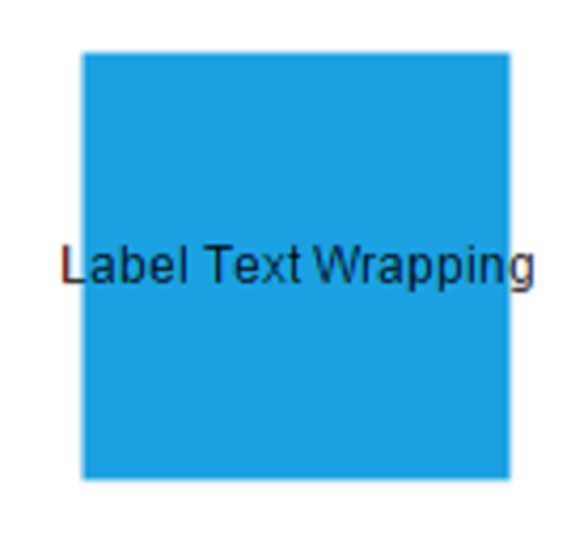Wrap Text-wrapping occurs, when the text overflows beyond the available node width.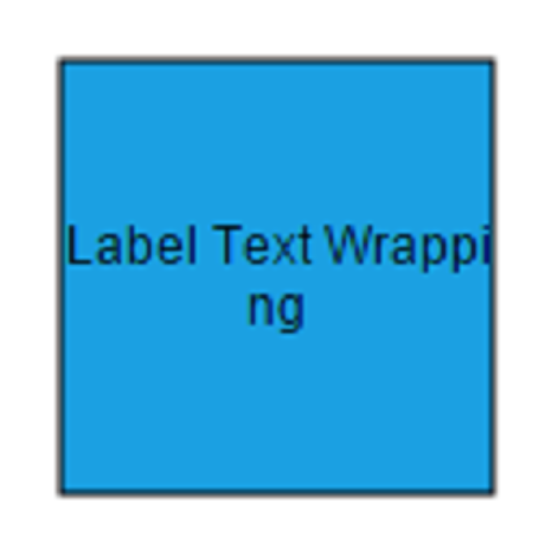WrapWithOverflow (Default) Text-wrapping occurs, when the text overflows beyond the available node width. However, the text may overflow beyond the node width in the case of a very long word.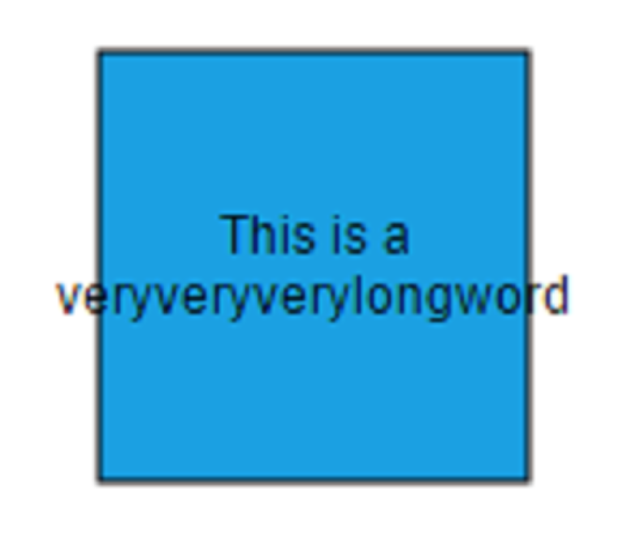## Text overflow

The label’s `TextOverflow` property is used control whether to display the overflowed content in node or not.

Source
Preview
index.tsx
``````import * as React from "react";
import * as ReactDOM from "react-dom";
import {
Diagram,
DiagramComponent,
NodeModel
} from "@syncfusion/ej2-react-diagrams";

// A node is created and stored in nodes array.
let node: NodeModel[] = [{
id: 'node1',
// Position of the node
offsetX: 100,
offsetY: 100,
// Size of the node
width: 100,
height: 100,
style: {
fill: '#6BA5D7',
strokeColor: 'white'
},
// Sets the annotation for the node
annotations: [{
content: 'Annotation Text',
// Sets the style for the text to be displayed
style: {
textOverflow: 'Ellipsis'
}
}]
}];

// initialize Diagram component

ReactDOM.render( <DiagramComponent id = "diagram"
width = {
'100%'
}
height = {
'600px'
}
nodes = {
node
}
// render initialized Diagram
/>,   document.getElementById("diagram") );``````

## Appearance

Source
Preview
index.tsx
``````import * as React from "react";
import * as ReactDOM from "react-dom";
import {
Diagram,
DiagramComponent,
NodeModel
} from "@syncfusion/ej2-react-diagrams";

// A node is created and stored in nodes array.
let node: NodeModel[] = [{
id: 'node1',
// Position of the node
offsetX: 100,
offsetY: 100,
// Size of the node
width: 100,
height: 100,
style: {
fill: '#6BA5D7',
strokeColor: 'white'
},
// Sets the annotation for the node
annotations: [{
content: 'Annotation Text',
// Sets the style for the text to be displayed
style: {
color: 'black',
bold: true,
italic: true,
fontSize: '12',
fontFamily: 'TimesNewRoman'
}
}]
}];

// initialize Diagram component

ReactDOM.render( <DiagramComponent id = "diagram"
width = {
'100%'
}
height = {
'600px'
}
nodes = {
node
}
// render initialized Diagram
/>,   document.getElementById("diagram") );``````

The fill, border, and opacity appearances of the text can also be customized with appearance specific properties of annotation. The following code illustrates how to customize background, opacity, and border of the annotation.

Source
Preview
index.tsx
``````import * as React from "react";
import * as ReactDOM from "react-dom";
import {
Diagram,
DiagramComponent,
NodeModel
} from "@syncfusion/ej2-react-diagrams";

// A node is created and stored in nodes array.
let node: NodeModel[] = [{
id: 'node1',
// Position of the node
offsetX: 100,
offsetY: 100,
// Size of the node
width: 100,
height: 100,
style: {
fill: '#6BA5D7',
strokeColor: 'white'
},
// Sets the annotation for the node
annotations: [{
content: 'Annotation Text',
style: {
color: 'black',
fill: 'white',
opacity: 0.7,
strokeColor: 'black',
strokeWidth: 2
}
}]
}];

// initialize Diagram component

ReactDOM.render( <DiagramComponent id = "diagram"
width = {
'100%'
}
height = {
'600px'
}
nodes = {
node
}
// render initialized Diagram
/>,   document.getElementById("diagram") );``````

## Interaction

Diagram allows annotation to be interacted by selecting, dragging, rotating, and resizing. Annotation interaction is disabled, by default. You can enable annotation interaction with the `constraints` property of annotation. You can also curtail the services of interaction by enabling either selecting, dragging, rotating, or resizing individually with the respective constraints property of annotation. The following code illustrates how to enable annotation interaction.

Source
Preview
index.tsx
``````import * as React from "react";
import * as ReactDOM from "react-dom";
import {
Diagram,
DiagramComponent,
NodeModel,
AnnotationConstraints
} from "@syncfusion/ej2-react-diagrams";

// A node is created and stored in nodes array.
let node: NodeModel[] = [{
id: 'node1',
// Position of the node
offsetX: 100,
offsetY: 100,
// Size of the node
width: 100,
height: 100,
style: {
fill: '#6BA5D7',
strokeColor: 'white'
},
// Sets the annotation for the node
annotations: [{
content: 'Annotation Text',
//Sets the constraints as Interaction
constraints: AnnotationConstraints.Interaction
}]
}];

// initialize Diagram component

ReactDOM.render( <DiagramComponent id = "diagram"
width = {
'100%'
}
height = {
'600px'
}
nodes = {
node
}
// render initialized Diagram
/>,   document.getElementById("diagram") );``````

## Edit

Diagram provides support to edit an annotation at runtime, either programmatically or interactively. By default, annotation is in view mode. But it can be brought to edit mode in two ways;

• Programmatically By using `startTextEdit` method, edit the text through programmatically.
• Interactively
• By double-clicking the annotation.
• By selecting the item and pressing the F2 key.

Double-clicking any annotation will enables editing and the node enables first annotation editing. When the focus of editor is lost, the annotation for the node is updated. When you double-click on the node/connector/diagram model, the `doubleClick` event gets triggered.

Diagram allows to create read-only annotations. You have to set the read-only property of annotation to enable/disable the read-only `constraints`. The following code illustrates how to enable read-only mode.

Source
Preview
index.tsx
``````import * as React from "react";
import * as ReactDOM from "react-dom";
import {
Diagram,
DiagramComponent,
NodeModel,
AnnotationConstraints
} from "@syncfusion/ej2-react-diagrams";

// A node is created and stored in nodes array.
let node: NodeModel[] = [{
id: 'node1',
// Position of the node
offsetX: 100,
offsetY: 100,
// Size of the node
width: 100,
height: 100,
style: {
fill: '#6BA5D7',
strokeColor: 'white'
},
// Sets the annotation for the node
annotations: [{
content: 'Annotation Text',
//Sets the constraints as Read only
}]
}];

// initialize Diagram component

ReactDOM.render( <DiagramComponent id = "diagram"
width = {
'100%'
}
height = {
'600px'
}
nodes = {
node
}
// render initialized Diagram
/>,   document.getElementById("diagram") );``````

## Drag Limit

• The diagram control now supports defining the `dragLimit` to the label while dragging from the connector and also update the position to the nearest segment offset.
• You can set the value to dragLimit `left`, `right`, `top`, and `bottom` properties which allow the dragging of connector labels to a certain limit based on the user defined values.
• By default, drag limit will be disabled for the connector. It can be enabled by setting connector constraints as drag.
• The following code illustrates how to set a dragLimit for connector annotations.
``````import * as React from "react";
import * as ReactDOM from "react-dom";
import {
Diagram,
DiagramComponent,
ConnectorModel,
AnnotationConstraints
} from "@syncfusion/ej2-react-diagrams";

// A node is created and stored in nodes array.
let connector: ConnectorModel[] = [{
id: 'connector2',
type: 'Orthogonal',
sourcePoint: { x: 300, y: 300 },
targetPoint: { x: 400, y: 400 },
annotations: [
{
content: 'connector1', offset:0.5,
//Enables drag constraints for a connector.
constraints:AnnotationConstraints.Interaction | AnnotationConstraints.Drag,
//Set drag limit for a connector annotation.
dragLimit:{left:20,right:20,top:20,bottom:20}
}
],
}];

// initialize Diagram component

ReactDOM.render( <DiagramComponent id = "diagram"
width = {
'100%'
}
height = {
'600px'
}
nodes = {
node
}
// render initialized Diagram
/>,   document.getElementById("diagram") );``````

## Multiple annotations

You can add any number of annotations to a node or connector. The following code illustrates how to add multiple annotations to a node.

Source
Preview
index.tsx
``````import * as React from "react";
import * as ReactDOM from "react-dom";
import {
Diagram,
DiagramComponent,
NodeModel,
AnnotationConstraints
} from "@syncfusion/ej2-react-diagrams";

// A node is created and stored in nodes array.
let node: NodeModel[] = [{
id: 'node1',
// Position of the node
offsetX: 100,
offsetY: 100,
// Size of the node
width: 100,
height: 100,
style: {
fill: '#6BA5D7',
strokeColor: 'white'
},
// Sets the multiple annotation for the node
annotations: [{
content: 'Left',
offset: {
x: 0.12,
y: 0.1
}
},
{
content: 'Center',
offset: {
x: 0.5,
y: 0.5
}
},
{
content: 'Right',
offset: {
x: 0.82,
y: 0.9
}
}
]
}];

// initialize Diagram component

ReactDOM.render( <DiagramComponent id = "diagram"
width = {
'100%'
}
height = {
'600px'
}